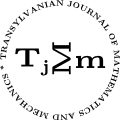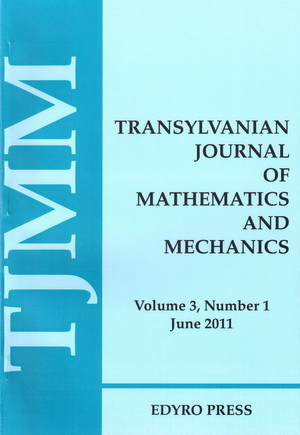ISSN: 2067-239X
ISSN(on-line): 2067-239X

Indexed in:
Mathematical Reviews
Zentralblatt MATH

Front cover# Volume 11 (2019), Number 1-2

## Certain Subclass of Meromorphic Functions Involving $q$-Ruscheweyh Differential Operator

### Author(s): ABDULLAH ALSOBOH and MASLINA DARUS

Abstract: In this paper, we introduce a new q-analogue of differential operator involving $q$-Ruscheweyh operator with a new subclass of meromorphic functions. We obtain coeﬃcient conditions and show some properties for function $f$ belonging to this subclass such as convolution conditions, closure and convex combinations. Finally, the neighbourhoods problem is solved.

## A Note on the Bessel Form of Parameter $3∕2$

### Author(s): BAGHDADI ALOUI and LOTFI KHÉRIJI

Abstract: In this manuscript, we consider a certain raising operator (with a nonzero free parameter) and we prove the following statement: up to normalization, the only orthogonal sequence that remain orthogonal after application of this raising operator is the one obtained by dilating the Bessel polynomials of parameter $3∕2$.

## Existence and Uniqueness Theorems for Fourth-Order Equations With Boundary Conditions

### Author(s): ROWAIDA ALRAJHI and LAZHAR BOUGOFFA

Abstract: The purpose of this paper is to prove the existence and uniqueness theorem of the boundary value problem for fourth-order differential equations ${u\left(4\right)}^{}+q\left(x\right)u\left(x\right)=g\left(x\right),\phantom{\rule{2.77695pt}{0ex}}0 subject to the BC: $u\left(0\right)={u}^{\prime }\left(0\right)={u}^{″}\left(1\right)={u}^{‴}\left(1\right)=0$ in the Sobolev space ${ℍ}^{4}\left(\left[0,1\right]\right)$ by using an a priori estimate. We also investigate the Schauder’s ﬁxed point theorem for proving the existence theorem of the boundary value problem for fourth-order nonlinear differential equations ${u}^{4}-f\left(x,{u}^{\prime },{u}^{\prime },{u}^{″},{u}^{‴}\right),\phantom{\rule{2.77695pt}{0ex}}0 under the above boundary conditions (BC), where $f:\left[0,1\right]×{ℝ}^{4}\to ℝ$ is a continuous function and satisfies $\left|f\left(x,u,v,w,z\right)\right|\le a+{a}_{0}\left|u\right|+{a}_{1}\left|v\right|+{a}_{2}\left|w\right|+{a}_{3}\left|z\right|,$ where $a,{a}_{i}>0,\phantom{\rule{2.77695pt}{0ex}}i=0,\dots ,3$.

## Hermite-Hadamard-Fejer Type Inequalities for $s-p-$Convex Functions of Several Kinds

### Author(s): AMBREEN ARSHAD and ASIF R. KHAN

Abstract: Earlier, Mehmet has done work on Hermite-Hadamard-Fejer type inequality for $p-$convex functions in . Now we generalized the work of Mehmet for $p-$convexity of Hermite-Hadamard-Fejer type inequalities for $s-p-$convex functions of 1st kind and 2nd kind.

## Positive Periodic Solutions for Second-Order Neutral Diﬀerence Equations With Variable Coefficients

### Author(s): ABDELOUAHEB ARDJOUNI and AHCENE DJOUDI

Abstract: In this article, we obtain sufficient conditions for the existence of positive periodic solutions for a second-order neutral difference equation with variable coefficients. The main tool employed here is the Krasnoselskii fixed point theorem dealing with a sum of two mappings, one is a contraction and the other is a completely continuous.

## Estimation of Upper Bounds Involving Katugampola Fractional Integrals

### Author(s): MUHAMMAD UZAIR AWAN, NOUSHEEN AKHTAR, MUHAMMAD ASLAM NOOR, MARCELA V. MIHAI and KHALIDA INAYAT NOOR

Abstract: The main objective of this article is to give some further generalizations of Hermite-Hadamard type inequalities via harmonic convex functions. The inequalities involve Katugampola’s fractional integral. We also give new estimation to upper bounds essentially using Katugampola’s fractional integrals. Special cases are also discussed.

## Generalizations of Opial Type Inequalities in Two Variables Using $p$-Norms

### Author(s): HÜSEYIN BUDAK

Abstract: In this paper, using the $p$-norms, we obtain some generalized Opial type inequalities in two variables for two functions. The results in this paper generalize several inequalities obtained in earlier works.

## Cubic Spline Approximation of the Reliability Polynomials of Two Dual Hammock Networks

### Author(s): GABRIELA CRISTESCU and VLAD-FLORIN DRĂGOI

Abstract: The property of preserving the convexity and concavity of the Bernstein polynomial and of the Bézier curves is used to generate a method of approximating the reliability polynomial of a hammock network. The mutual behaviour of the reliability polynomials of two dual hammock networks is used to generate a system of constraints since the initial information is not enough for using a classical approximation scheme. A cubic spline function is constructed to generate approximations of the coefficients of the two reliability polynomials. As consequence, an approximation algorithm is described and tested through simulations on hammocks with known reliability, comparing the results with the results of approximations attempts from literature.

## A Note on Numerical Comparison of Some Multiplicative Bounds Related to Weighted Arithmetic and Geometric Means

### Author(s): SILVESTRU SEVER DRAGOMIR and ALASDAIR MCANDREW

Abstract: In this note we provided some numerical comparison for the upper and lower bounds in some recent inequalities related to the famous Young’s inequality for two positive numbers. We drew the conclusion that neither of the inequalities below is always best.

## Some Discrete Inequalities for Convex Functions Deﬁned on Linear Spaces

### Author(s): SILVESTRU SEVER DRAGOMIR

Abstract: In this paper we provide some discrete inequalities related to the Hermite-Hadamard result for convex functions deﬁned on convex subsets in a linear space. Applications for norms and univariate real functions with an example for the logarithm, are also given.

## Extension of Results Analogous to Beta Functions by the Way of $\left(\alpha ,s\right)$ Pre Invexity

### Author(s): ZAMIR HUSSAIN and MUHAMMAD MUDDASSAR

Abstract: In this note, we establish an extension of existing results analogous to Euler Beta function link with the integral inequalities of the type of Hermite-Hadamard’s by weaken the condition of convexity, when the power of the absolute value is $\left(\alpha ,s\right)$ preinvex mappings.

## On Some Connected Groups of Automorphisms of Weil Algebras

### Author(s): MIROSLAV KUREŠ and JAN ŠÚTORA

Abstract: The method of direct calculation of the group of $ℝ$-algebra automorphisms of a Weil algebra is presented in detail. The paper is focused on the case of a one-componental group and presents two cases of values of the determinant of its linear part.

## Differential Subordinations Defined by Using Sălăgean Integral Operator at the Class of Meromorphic Functions

### Author(s): CAMELIA MĂDĂLINA MARTINESCU and SORIN MIREL STOIAN

Abstract: By using the Sălăgean integral operator ${I}^{n}f\left(z\right),\phantom{\rule{2.77695pt}{0ex}}z\in U$, we introduce a general class of holomorphic functions denoted by ${\sum }_{k,m}\left(\alpha ,n\right)$ and we obtain an inclusion relation related to this class and some differential subordinations.

## On Certain Family of Multivalent Harmonic Functions Associated With Jung–Kim–Srivastava Operator

Abstract: New families of harmonic multivalent functions in terms Jung–Kim–Srivastava integral operator are introduced. Sufficient and necessary conditions for coefficients are obtained. Also extreme points, distortion bounds and convex combinations are investigated.

## Exponentially General Convex Functions

### Author(s): MUHAMMAD ASLAM NOOR and KHALIDA INAYAT NOOR

Abstract: In this paper, we define and introduce some new classes of the exponentially convex functions involving an arbitrary function, which is called the exponentially general convex function. We investigate several properties of the exponentially general convex functions and discuss their relations with convex functions. Optimality conditions are characterized by a class of variational inequalities, which is called the exponentially general variational inequality. Several new results characterizing the exponentially general convex functions are obtained. Results obtained in this paper can be viewed as significant improvement of previously known results.

## A Finite Difference Method for Solution of Nonlinear Two Point Boundary Value Problem With a Neumann Boundary Conditions

### Author(s): PRAMOD KUMAR PANDEY and FAISAL AL-SHOWAIKH

Abstract: A finite difference scheme for the solution of two point boundary value problem in ordinary differential equations subject to the Neumann boundary conditions presented in this article. The propose scheme is tested on linear and non-linear problems. The solution of the discretized problems was solved by iterative methods, i.e. Gauss-Seidel and Newton-Raphson method. The computational results demonstrate reliability and efficiency of the developed finite difference method. Moreover, numerical results confirm that scheme has second order accuracy.

## Opial Type Inequalities for Conformable Fractional Integrals via Convexity

### Author(s): MEHMET ZEKI SARIKAYA and CANDAN CAN BILISIK

Abstract: The main target addressed in this article are presenting Opial type inequalities for Katugampola conformable fractional integral. In accordance with this purpose we try to use more general type of function in order to make a generalization. Thus our results cover the previous published studies for Opial type inequalities.

## On Some Common Fixed Points Theorems in Intuitionistic Fuzzy Metric Spaces and Its Applications

### Author(s): RAJINDER SHARMA and DEEPTI THAKUR

Abstract: In this paper, we established some common fixed point theorems for pairs of semi compatible and occasionally weakly compatible mappings in an intuitionistic fuzzy metric space (briefly IFM space) satisfying contractive type condition. In this paper, we observe that the notion of common property (E.A.) relatively relaxes the required containment of the range of one mapping into the range of other which is utilized to construct the sequence of joint iterates. We extended the results established in  to intuitionistic fuzzy metric space.

## New Representations of Integrals of Polylogarithmic Functions With Quadratic Argument

### Author(s): A. SOFO and G. SORRENTINO

Abstract: In this paper we explore the representation and many connections between integrals of products of polylogarithmic functions with a quadratic argument and Euler sums. The connection between polylogarithmic functions and Euler sums is well known. Some examples of integrals of products of polylogarithmic functions in terms of Riemann zeta values and Dirichlet values will be given.

## Voronec Equations for Nonlinear Nonholonomic Systems

### Author(s): FEDERICO TALAMUCCI

Abstract: One of the founders of the mechanics of nonoholonomic systems is Voronec who published in 1901 a significant generalization of the Čaplygin’s equations, by removing some restrictive assumptions. In the frame of nonholonomic systems, the Voronec equations are probably less frequent and common with respect to the prevalent methods of quasi–coordinates (Hamel–Boltzmann equations) and of the acceleration energy (Gibbs–Appell equations). In this paper we start from the case of linear nonholonomic constraints, in order to extend the Voronec equations to nonlinear nonholonomic systems. The comparison between two ways of expressing the equations of motion is performed. We ﬁnally comment that the adopted procedure is appropriated to implement further extensions.

Abstract: The aim of the present paper is to introduce a class ${\sum }_{p}^{\ast }\left(A,B,\mu ,𝜃\right)$ of meromorphic univalent functions in $E=\left\{0<\left|z\right|<1\right\}$ and investigate coefficient estimates, distortion properties and radius of convexity estimates for this class. Furthermore, it is shown that the class ${\sum }_{p}^{\ast }\left(A,B,\mu ,𝜃\right)$ is closed under convex linear combinations, convolutions and integral transforms.